### 2 随机森林

#### 2.1 随机森林原理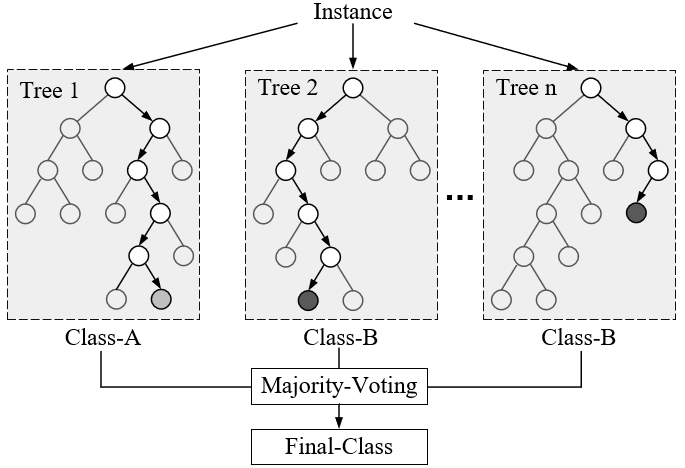#### 2.2 随机森林示例代码

1def __init__(self,2             n_estimators=100,3             criterion="gini",4             max_depth=None,5             min_samples_split=2,6             min_samples_leaf=1,7             max_features="auto",8             bootstrap=True,9             max_samples=None):

xxxxxxxxxx61if __name__ == '__main__':2    x_train, x_test, y_train, y_test = load_data()3    model = RandomForestClassifier(n_estimators=2, max_features=3, 4                                   random_state=2)5    model.fit(x_train, y_train)6    print(model.score(x_test, y_test)) # 0.95

### 3 特征重要性评估

#### 3.1 决策树中的特征评估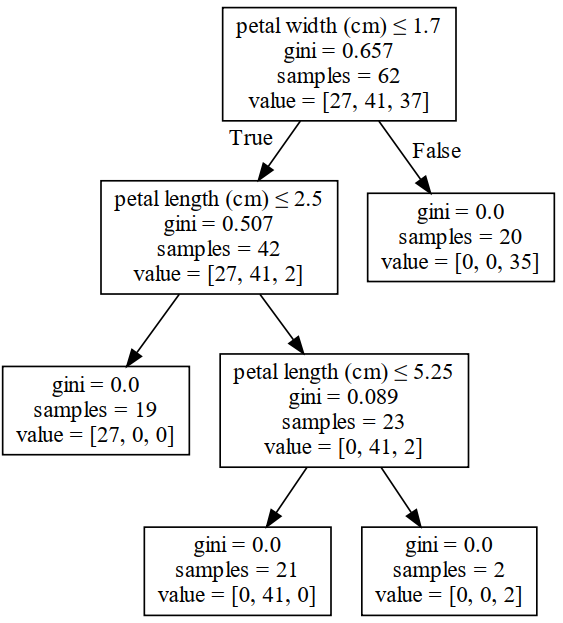#### 3.2 随机森林中的特征评估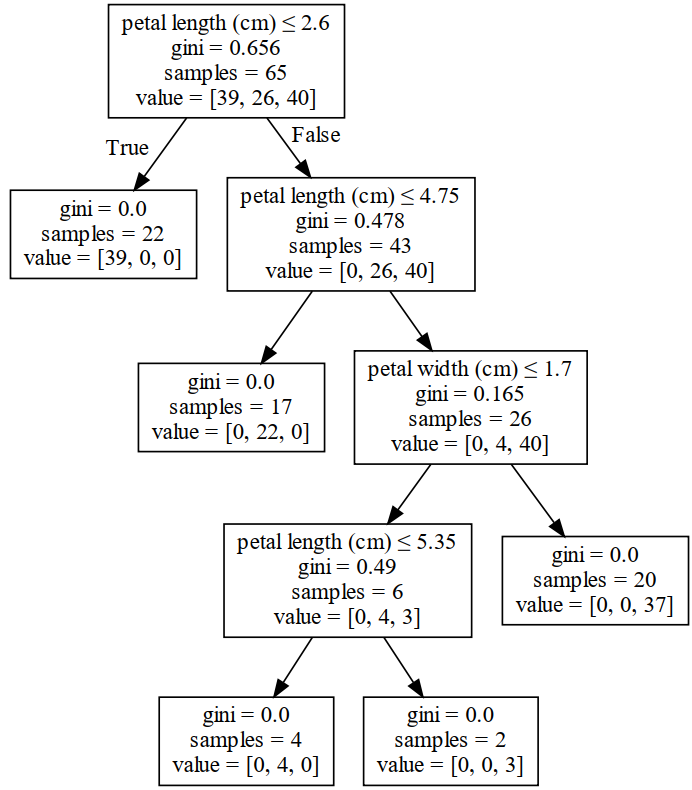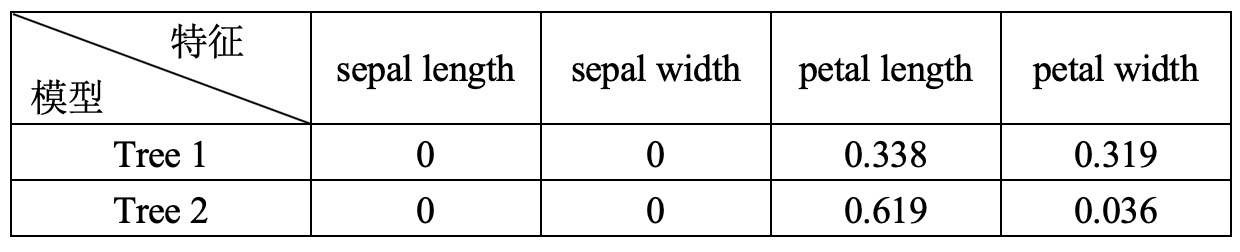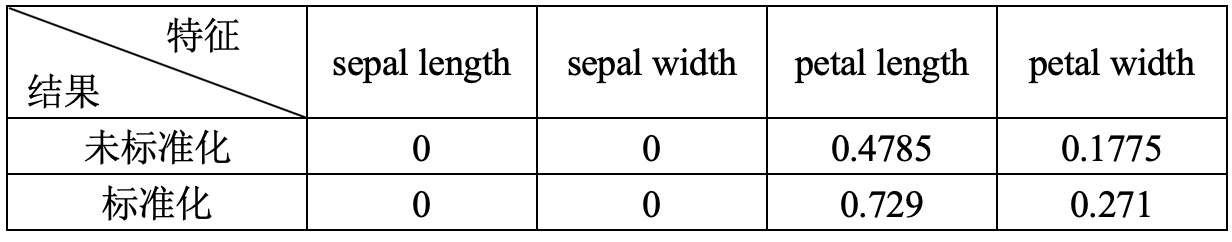### 相关阅读

 决策树的生成之ID3与C4.5

 集成模型：Bagging、Boosting和Stacking

 随机森林在sklearn中的使用